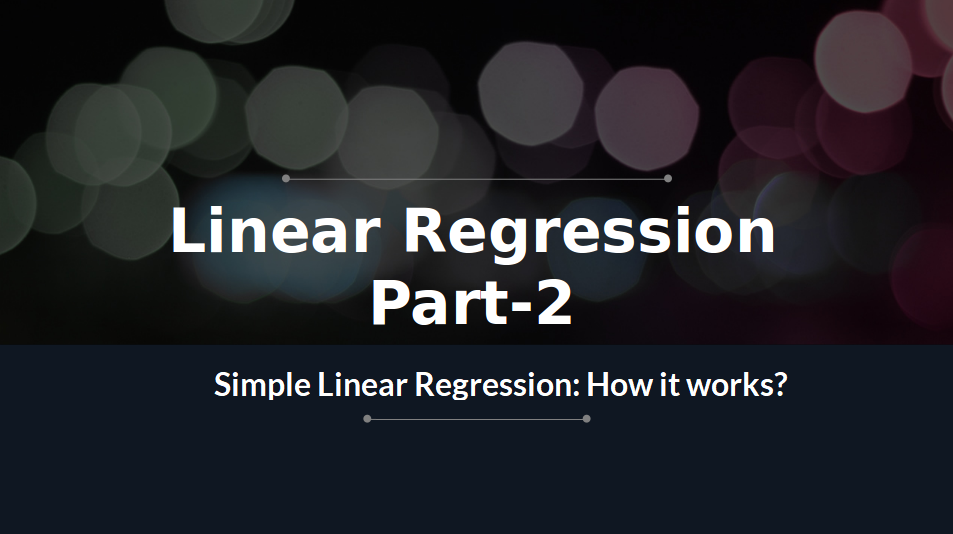## Simple Linear Regression: How It works? (Python Implementation)Simple Linear Regression: How It works? (Python Implementation)

# Linear Regression (Python Implementation)

This article discusses the basics of linear regression and its implementation in Python programming language.
Linear regression is a statistical approach for modelling the relationship between a dependent variable with a given set of independent variables.
Note: In this article, we refer dependent variables as response and independent variables as features for simplicity.
In order to provide a basic understanding of linear regression, we start with the most basic version of linear regression, i.e. Simple linear regression.

## Simple Linear Regression

Simple linear regression is an approach for predicting a response using a single feature.

It is assumed that the two variables are linearly related. Hence, we try to find a linear function that predicts the response value(y) as accurately as possible as a function of the feature or independent variable(x).
Let us consider a dataset where we have a value of response y for every feature x:For generality, we define:
x as feature vector, i.e x = [x_1, x_2, …., x_n],
y as response vector, i.e y = [y_1, y_2, …., y_n]
for n observations (in above example, n=10).
A scatter plot of above dataset looks like:-Now, the task is to find a line which fits best in above scatter plot so that we can predict the response for any new feature values. (i.e a value of x not present in the dataset)

This line is called the regression line.
The equation of the regression line is represented as:Here,
• h(x_i) represents the predicted response value for ith observation.
• b_0 and b_1 are regression coefficients and represent y-intercept and slope of regression line respectively.
To create our model, we must “learn” or estimate the values of regression coefficients b_0 and b_1. And once we’ve estimated these coefficients, we can use the model to predict responses!
In this article, we are going to use the Least Squares technique.
Now consider:Here, e_i is a residual error in ith observation.
So, our aim is to minimize the total residual error.
We define the squared error or cost function, J as:and our task is to find the value of b_0 and b_1 for which J(b_0,b_1) is minimum!
Without going into the mathematical details, we present the result here:where SS_xy is the sum of cross-deviations of y and x:and SS_xx is the sum of squared deviations of x:Note: The complete derivation for finding least squares estimates in simple linear regression can be found here.
Given below is the python implementation of the above technique on our small dataset:
 import numpy as np  import matplotlib.pyplot as plt     def estimate_coef(x, y):      # number of observations/points      n = np.size(x)         # mean of x and y vector      m_x, m_y = np.mean(x), np.mean(y)         # calculating cross-deviation and deviation about x      SS_xy = np.sum(y*x) - n*m_y*m_x      SS_xx = np.sum(x*x) - n*m_x*m_x         # calculating regression coefficients      b_1 = SS_xy / SS_xx      b_0 = m_y - b_1*m_x         return(b_0, b_1)     def plot_regression_line(x, y, b):      # plotting the actual points as scatter plot      plt.scatter(x, y, color = "m",                 marker = "o", s = 30)         # predicted response vector      y_pred = b + b*x         # plotting the regression line      plt.plot(x, y_pred, color = "g")         # putting labels      plt.xlabel('x')      plt.ylabel('y')         # function to show plot      plt.show()     def main():      # observations      x = np.array([0, 1, 2, 3, 4, 5, 6, 7, 8, 9])      y = np.array([1, 3, 2, 5, 7, 8, 8, 9, 10, 12])         # estimating coefficients      b = estimate_coef(x, y)      print("Estimated coefficients:\nb_0 = {}  \            \nb_1 = {}".format(b, b))         # plotting regression line      plot_regression_line(x, y, b)     if __name__ == "__main__":      main()
The output of the above piece of code is:
Estimated coefficients:
b_0 = -0.0586206896552
b_1 = 1.45747126437

And the graph obtained looks like this:## http://bit.ly/2Ufe34U

1.1.The development of artificial intelligence (AI) has propelled more programming architects, information scientists, and different experts to investigate the plausibility of a vocation in machine learning. Notwithstanding, a few newcomers will in general spotlight a lot on hypothesis and insufficient on commonsense application. machine learning projects for final year In case you will succeed, you have to begin building machine learning projects in the near future.

Projects assist you with improving your applied ML skills rapidly while allowing you to investigate an intriguing point. Furthermore, you can include projects into your portfolio, making it simpler to get a vocation, discover cool profession openings, and Final Year Project Centers in Chennai even arrange a more significant compensation.

Data analytics is the study of dissecting crude data so as to make decisions about that data. Data analytics advances and procedures are generally utilized in business ventures to empower associations to settle on progressively Python Training in Chennai educated business choices. In the present worldwide commercial center, it isn't sufficient to assemble data and do the math; you should realize how to apply that data to genuine situations such that will affect conduct. In the program you will initially gain proficiency with the specialized skills, including R and Python dialects most usually utilized in data analytics programming and usage; Python Training in Chennai at that point center around the commonsense application, in view of genuine business issues in a scope of industry segments, for example, wellbeing, promoting and account.

The Nodejs Projects Angular Training covers a wide range of topics including Components, Angular Directives, Angular Services, Pipes, security fundamentals, Routing, and Angular programmability. The new Angular TRaining will lay the foundation you need to specialise in Single Page Application developer. Angular Training

2.3.for your estimated coefficients I seem to be getting different figures
b_0 = 1.2363636363636363
b_1 = 1.1696969696969697

1.It will vary with the system configuration

2.Ok I get you, I further used excel linear regression plotting and got the same result like I got in python